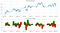# Evaluate Price Oscillator Stock Trading Strategy

## Algorithmic Trading and PnL EvaluationImage by Author
`def SPFutures():   df = pd.read_csv("July_Futures_15min.csv")  df.set_index('timestamp', inplace=True)  df.sort_index(ascending=True, inplace=True)  df.index = pd.to_datetime(df.index)  df = df[df['volume'] > 0]  df.sort_index(ascending=True, inplace=True)   df.drop_duplicates(inplace=True) # dropping duplicates if any  df.fillna(method='pad', inplace=True)   return (df)df = SPFutures()print(df.head())`

# Stochastic Oscillator:

`d = df.copy()# Create the "L14" column in the DataFramed['L_14'] = d['es_low'].rolling(window=14).min()#Create the "H14" column in the DataFramed['H_14'] = d['es_high'].rolling(window=14).max()#Create the "%K" column in the DataFramed['%K'] = 100*((d['es_close'] - d['L_14']) / (d['H_14'] - d['L_14']))#Create the "%D" column in the DataFramed['%D'] = d['%K'].rolling(window=3).mean()#  plotfig, axes = plt.subplots(nrows=2, ncols=1,figsize=(15,10))d['es_close'].plot(ax=axes); axes.set_title('Close')d[['%K','%D']].plot(ax=axes); axes.set_title('Oscillator')plt.tight_layout(); plt.show()`
`d['enter_sell'] = ((d['%K'] < d['%D']) & (d['%K'].shift(1) > d['%D'].shift(1))) & (d['%D'] > 75)d['exit_sell'] = ((d['%K'] > d['%D']) & (d['%K'].shift(1) < d['%D'].shift(1)))d['short'] = np.nand.loc[d['enter_sell'],'short'] = -1d.loc[d['exit_sell'],'short'] = 0#Set initial position on day 1 to flatd['short'] = 0d['short'] = d[short'].fillna(method='pad')d['enter_buy'] = ((d['%K'] > d['%D']) & (d['%K'].shift(1) < d['%D'].shift(1))) & (d['%D'] < 25)d['exit_buy'] = ((d['%K'] < d['%D']) & (d['%K'].shift(1) > d['%D'].shift(1)))d['long'] = np.nand.loc[d['enter_buy'],'long'] = 1d.loc[d['exit_buy'],'long'] = 0d['long'] = 0d['long'] = d['long'].fillna(method='pad')# Add Long and Short positions together to get final strategy position (1 for long, -1 for short and 0 for flat)d['Position'] = d['long'] + d['short']`

## Visualization:

`d['market_returns'] = d['es_close'].pct_change()d['strategy_returns'] = d['market_returns'] * d['Position'].shift(1)d[['strategy_returns','market_returns']].cumsum().plot()plt.show()`

# Calculating Price Oscillator:

Tradition and convention have deemed 26 days to be the dividing line between the short term and the “minor intermediate” term in the stock market, with the “very short” term lasting between five and 13 days.(Investopedia)

## Parameters for EMA Calculation:

`fastPeriod = 10 fastSmooth = 2 / (fastPeriod + 1) fastEma = 0fastEma_val = [] slowPeriod = 40 slowSmooth = 2 / (slowPeriod + 1) slowEma = 0slowEma_val = [] poValues = [] `
`def ema(price, period):  ema = price.rolling(period).mean()  return emadef po(price, period1, period2):  median = price.rolling(2).median()  short = ema(median, period1)  long = ema(median, period2)  po = short - long  po_df = DataFrame(po).rename(columns = {'Close':'po'})  return po_dfdf['po'] = po(df['es_close'], 10, 40)df.dropna(inplace=True)plt.figure(figsize=(15,8))ax1 = plt.subplot2grid((10,1), (0,0), rowspan = 5, colspan = 1)ax2 = plt.subplot2grid((10,1), (6,0), rowspan = 4, colspan = 1)ax1.plot(df['es_close'])ax1.set_title('S&P E-Mini closing price')for i in range(len(df)):  if df['po'][i-1] > df['po'][i]:    ax2.bar(df.index[i], df['po'][i], color = 'green')  else:    ax2.bar(df.index[i], df['po'][i], color = 'red')ax2.set_title('S&P E-mini Price Oscillator')plt.show()`

## Trading strategy:

`orders = [] positions = [] pnls = [] last_buyPrice = 0 last_sellPrice = 0 position = 0 buy_sumPrice_qty = 0 buy_sum_qty = 0 sell_sumPrice_qty = 0 sell_sum_qty = 0 open_pnl = 0 closed_pnl = 0 `
`po_buyEnter = -10 po_sellEnter = 10 min_price_movement_from_last_trade = 10 num_stocks_per_trade = 10 min_profit_to_close = 10 * num_stocks_per_trade `
`maPeriods = 20 priceHist = [] close = df['es_close'].copy()for close_price in close:  priceHist.append(close_price)  if len(priceHist) > maPeriods:     del (priceHist)  sma = stats.mean(priceHist)  variance = 0   for histPrice in priceHist:    variance = variance + ((histPrice - sma) ** 2)   stdev = math.sqrt(variance / len(priceHist))  stdev_factor = stdev/15  if stdev_factor == 0:    stdev_factor = 1  if (fastEma == 0):     fastEma = close_price    slowEma = close_price  else:    fastEma = (close_price - fastEma) * smooth_fast * stdev_factor + fastEma    slowEma = (close_price - slowEma) * smooth_slow * stdev_factor + slowEma  fastEma_val.append(fastEma)  slowEma_val.append(slowEma)  po = fastEma - slowEma  poValues.append(po)  if ((po > po_sellEnter * stdev_factor and abs(close_price - last_sellPrice) > min_price_movement_from_last_trade * stdev_factor)  or  (position > 0 and (po >= 0 or open_pnl > min_profit_to_close / stdev_factor))):   orders.append(-1) # sell trade  last_sellPrice = close_price  position -= num_stocks_per_trade   sell_sumPrice_qty += (close_price * num_stocks_per_trade) # update vwap sell-price  sell_sum_qty += num_stocks_per_trade  print( "SELL ", num_stocks_per_trade, " @ ", close_price, "Position => ", position)  elif ((po < po_buyEnter * stdev_factor and abs(close_price - last_buyPrice) > min_price_movement_from_last_trade * stdev_factor)       or  (position < 0 and (po <= 0 or open_pnl > min_profit_to_close / stdev_factor))):   orders.append(+1) # buy trade  last_buyPrice = close_price  position += num_stocks_per_trade # increase position by the size of this trade  buy_sumPrice_qty += (close_price * num_stocks_per_trade) # update the vwap buy-price  buy_sum_qty += num_stocks_per_trade  print( "BUY ", num_stocks_per_trade, " @ ", close_price, "POSITION: ", position ); print()  else:  orders.append(0)  positions.append(position)  open_pnl = 0  if position > 0:    if sell_sum_qty > 0:       open_pnl = abs(sell_sum_qty) * (sell_sumPrice_qty/sell_sum_qty - buy_sumPrice_qty/buy_sum_qty)    open_pnl += abs(sell_sum_qty - position) * (close_price - buy_sumPrice_qty / buy_sum_qty)  elif position < 0:    if buy_sum_qty > 0:       open_pnl = abs(buy_sum_qty) * (sell_sumPrice_qty/sell_sum_qty - buy_sumPrice_qty/buy_sum_qty)    open_pnl += abs(buy_sum_qty - position) * (sell_sumPrice_qty/sell_sum_qty - close_price)  else:    closed_pnl += (sell_sumPrice_qty - buy_sumPrice_qty)    buy_sumPrice_qty = 0    buy_sum_qty = 0    sell_sumPrice_qty = 0    sell_sum_qty = 0    last_buyPrice = 0    last_sellPrice = 0  print( "Open/PnL-> ", open_pnl, " Closed/PnL-> ", closed_pnl, " Total/PnL-> ", (open_pnl + closed_pnl) );  pnls.append(closed_pnl + open_pnl)`
`data = df.copy()data = data.assign(closePrice = pd.Series(close, index =df.index))data = data.assign(short = pd.Series(fastEma_val, index = df.index))data = data.assign(long = pd.Series(slowEma_val, index = df.index))data = data.assign(priceOs = pd.Series(poValues, index = df.index))data = data.assign(trades = pd.Series(orders, index = data.index))data = data.assign(position = pd.Series(positions, index = data.index))data = data.assign(pnl = pd.Series(pnls, index=data.index))data = data[['closePrice','short','long','priceOs','trades','position','pnl']]data.head(2)`
`plt.figure(figsize = (10, 6))data['closePrice'].plot(color = 'gray',lw = 1., legend=True)data['short'].plot(color ='green', lw = 1., legend=True)data['long'].plot(color ='red', lw = 1., legend=True)plt.title("E-Mini S &P 500 Futures - Market price")plt.show()`
`plt.figure(figsize = (15, 6))data['priceOs'].plot(color='gray', lw=2., legend=True)plt.plot(data.loc[data.trades == 1 ].index,data.priceOs[data.trades == 1 ], color='r', lw=0, marker='^', markersize=7, label='buy')plt.plot(data.loc[data.trades == -1 ].index,data.priceOs[data.trades == -1 ], color='green', lw=0, marker='v', markersize=7, label='sell')plt.axhline(y=0, lw=0.5, color='k')for i in range(po_buyEnter, po_buyEnter * 5, po_buyEnter ):  plt.axhline(y=i, lw=1., color='r')for i in range(po_sellEnter, po_sellEnter * 5, po_sellEnter ):  plt.axhline(y=i, lw=1., color='green')plt.legend()plt.title('E-Mini S&P 500 Futures-Price Oscillator based Buy/Sell trading signals')plt.show()`
`plt.figure(figsize = (15, 6))data['pnl'].plot(color='k', lw=1., legend=True)plt.plot(data.loc[data.pnl > 1 ].index, data.pnl[data.pnl > 1 ], color='green', lw=0, marker='.')plt.plot(data.loc[data.pnl < 1 ].index, data.pnl[data.pnl < 1 ], color='r', lw=0, marker='.')plt.legend()plt.title('P&Ls achieved by experimental strategy')plt.show()`

# Key takeaways:

Data Science Practice Lead at KSG Analytics Pvt. Ltd.

## More from Sarit Maitra

Data Science Practice Lead at KSG Analytics Pvt. Ltd.